Yao, L., and Li, Y. (2023). “Simulation study on static characteristics of Qing-style ‘one bucket three liters’ column-cap Dou-Gong bracket,” BioResources 18(4), 6955-6970.

#### Abstract

The Dou-Gong bracket is a key transition component between the roof and the column in ancient Chinese wooden structures. In this paper, the static structural behavior of Qing-style ‘one bucket three liters’ Column-cap Dou-Gong Bracket (third-class material) full-scale test model was studied by simulation. The simulation of monotonic static loading was carried out in the vertical (Z-axis) direction, and the simulation of quasi-static loading under horizontal low-cycle reciprocating load was carried out in the horizontal direction (Y-axis, X-axis). The material of the simulation was P. sylvestris, and the setting of its material parameters referred to the results obtained from the material property tests. An anisotropic constitutive model suitable for the properties of wood materials was selected, and the elastoplasticity of wood in the simulation was defined by the Hill yield rule. The static structural behavior of the component in the Z-axis could be described by the variable stiffness linear elastic mechanical model. The static structural behavior of the component in the Y-axis and X-axis could be described by a multi-linear restoring force model. According to the simulation results, the key mechanical indexes of Dou-Gong bracket component in the Z, Y, and X axes were calculated from three aspects of strength, deformation, and energy.

Simulation Study on Static Characteristics of Qing-style ‘One Bucket Three Liters’ Column-cap Dou-Gong Bracket

Lihong Yao * and Yuanhe Li

The Dou-Gong bracket is a key transition component between the roof and the column in ancient Chinese wooden structures. In this paper, the static structural behavior of Qing-style ‘one bucket three liters’ Column-cap Dou-Gong Bracket (third-class material) full-scale test model was studied by simulation. The simulation of monotonic static loading was carried out in the vertical (Z-axis) direction, and the simulation of quasi-static loading under horizontal low-cycle reciprocating load was carried out in the horizontal direction (Y-axis, X-axis). The material of the simulation was P. sylvestris, and the setting of its material parameters referred to the results obtained from the material property tests. An anisotropic constitutive model suitable for the properties of wood materials was selected, and the elastoplasticity of wood in the simulation was defined by the Hill yield rule. The static structural behavior of the component in the Z-axis could be described by the variable stiffness linear elastic mechanical model. The static structural behavior of the component in the Y-axis and X-axis could be described by a multi-linear restoring force model. According to the simulation results, the key mechanical indexes of Dou-Gong bracket component in the Z, Y, and X axes were calculated from three aspects of strength, deformation, and energy.

DOI: 10.15376/biores.18.4.6955-6970

Keywords: Dou-Gong bracket; Wood component; Static characteristics; Finite element simulation; Pinus sylvestris

Contact information: College of Material Science and Art Design, Inner Mongolia Agricultural University, Hohhot 010018, P.R. China; *Corresponding author: yaolihong82@163.com

Lihong Yao and Yuanhe Li contributed equally to this work.

INTRODUCTION

The Dou-Gong bracket is a unique component in ancient Chinese wooden structures (Fang et al. 2001; Xue et al. 2016), located in between the roof and the column. Its structural role is to increase the distance from the eaves of the roof truss, reduce the span of the beam, and transfer all the loads of the roof to the column (Meng et al. 2018). The configuration of Dou-Gong bracket is complex (Sha et al. 2021), with a large number of components with mortise and tenon technology (D’Ayala and Tsai 2008) and an independent modulus system. Dou-Gong plays an important role in the ancient Chinese wood structure building system (Wu et al. 2018), and it is important to understand the scientific and technological connotation of Dou-Gong. The most representative Dou-Gong brackets in ancient Chinese wooden structure buildings are divided into two categories: Song-style and Qing-style Dou-Gong brackets. The production of Qing-style Dou-Gong brackets follow a stricter normative system, among which the ‘one bucket and three liters’ column-cap Dou-Gong bracket is the most basic and representative form of Qing-style Dou-Gong brackets.

The invention motivation of Dou-Gong bracket is caused by many factors. First of all, its modulus system is the standard for all components to be drawn and made. Secondly, the application of mortise and tenon technology is convenient for the demolition, relocation, and repair of wood structure buildings; in addition, the invention of Dou-Gong bracket makes it possible to use only one material of wood to realize the construction of the whole wooden frame and present a unique roof structure form under the limited technical conditions in ancient times.

According to the provisions of the modulus system in Engineering Practice Regulations of the Qing Dynasty, the Dou-Gong bracket is divided into 11 different grades according to the size, and each grade has different scale factors, which are applicable to buildings of different sizes. The research object of this paper comes from the provisions of the Engineering Practice Regulations of the Qing Dynasty. The Engineering Practice Regulations clearly stipulates the structural form of the first to eleventh-class timber and the detailed dimensions of each component in the Dou-Gong bracket. The object of this study is third-class timber, of which the scale factor is equal to 16 mm. The perspective view and explosion diagram of the ‘one bucket three liters’ Column-cap Dou-Gong bracket test model of the third-class are shown in Fig. 1. In the Dou-Gong component, there are a total of 15 units including 12 main parts and 3 wood pins, which are divided into two types.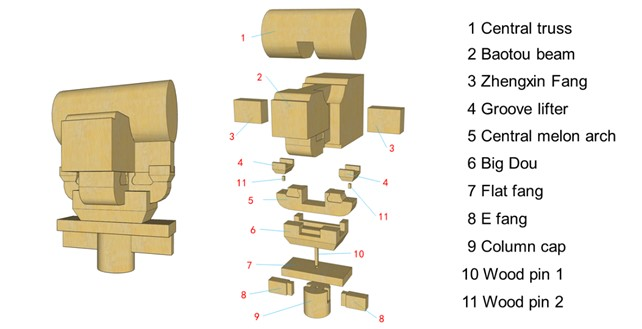Fig. 1. The perspective view (left) and explosion diagram (right) of the ‘one bucket three liters’ column-cap Dou-Gong bracket test model

In the traditional wood structure test, the production cost of the full-scale model of Dou-Gong bracket is high (Chen et al. 2018; Meng et al. 2019). Only the measuring points with the measuring device can obtain the test results, and there are a lot of uncontrollable errors in the structural test, which has certain limitations. The simulation technology based on finite element analysis is effective in analyzing the static characteristics of Dou-Gong bracket after years of development and a large number of structural tests (Suzuki and Maeno 2006; Bedon et al. 2015; Wu et al. 2020). Especially in the static behavior of full-scale models (Sciomenta et al. 2018), it avoids the high cost of specimen production and obtains research results in a targeted manner.

In this paper, the static structural behavior of the refined model of the whole structure of Qing-style ‘one bucket three liters’ Column-cap Dou-Gong Bracket was systematically simulated by using ANSYS finite element software. The simulation results of the key mechanical indexes of Dou-Gong Bracket were obtained from three aspects: strength, deformation, and energy.

EXPERIMENTAL

Measurement of Basic Mechanical Parameters of P. sylvestris

The material set in the simulation was P. sylvestris; hence, the air-dry density, elastic modulus, shear modulus, Poisson’s ratio, flexural strength, compressive strength parallel to grain, and compressive strength perpendicular to grain of P. sylvestris at 12% moisture content were measured in the material property test. According to GB/T 1933 (2009), the air-dried density of P. sylvestris at 12% moisture content was 0.493 g/cm3. According to GB/T 15777 (2017), GB/T 1943 (2009), and LY/T 3297 (2022), the elastic modulus, Poisson’s ratio, and shear modulus of P. sylvestris at 12% moisture content were measured by resistance strain method, and the test results are shown in Table 1. According to GB/T 1935 (2009), GB/T 1939 (2009), and GB/T 1936.1 (2009), the compressive strength parallel to grain of P. sylvestris wood was 35.2 MPa, the compressive strength perpendicular to grain was 5.14 MPa, and the bending strength was 52.9 MPa. Most of the components in Dou-Gong bracket are compressed by the transverse grain of wood. The wood texture of some components is randomly adjusted according to the material, which avoids the serious wood defects such as knots and decay.

Table 1. Elastic Modulus, Poisson’s Ratio, and Shear Modulus of P. sylvestris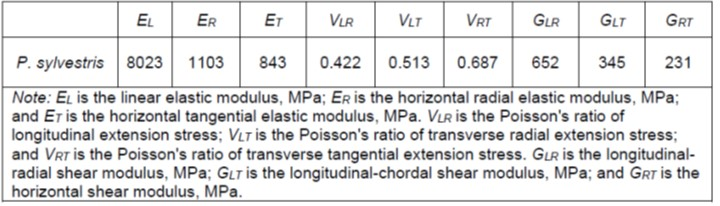Material Parameter Setting and Wood Constitutive Model in Simulation

The simulation of Dou-Gong bracket was based on the finite element analysis of ANSYS (ANSYS workbench 2021 R1; ANSYS, Inc, San Diego, USA), of which the material parameter setting in the simulation referred to the results of material test. The plasticity and elasticity of the material were defined according to the stress/strain curve obtained from the test and the Hill yield criterion. The stress / strain curves of the longitudinal, transverse radial, and transverse chord of P. sylvestris after fitting according to the material test data are shown in Fig. 2. The wood constitutive model (Fig. 3) was orthotropic, such that the three symmetry planes of this three-dimensional orthotropic material could directly specify the elastic matrix, using a total of 9 independent elastic coefficients. The material model could be defined by filling the elastic matrix coefficients in ANSYS.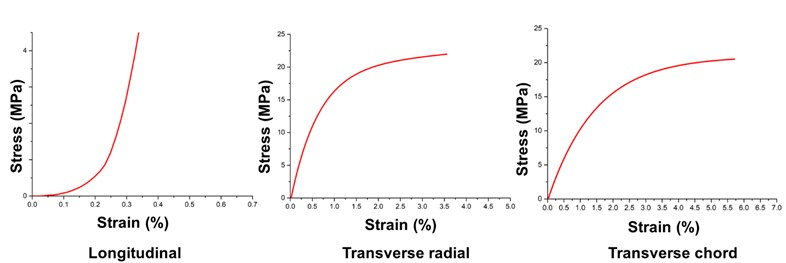Fig. 2. Stress/strain curves of the longitudinal, transverse radial, and transverse chord of P. sylvestris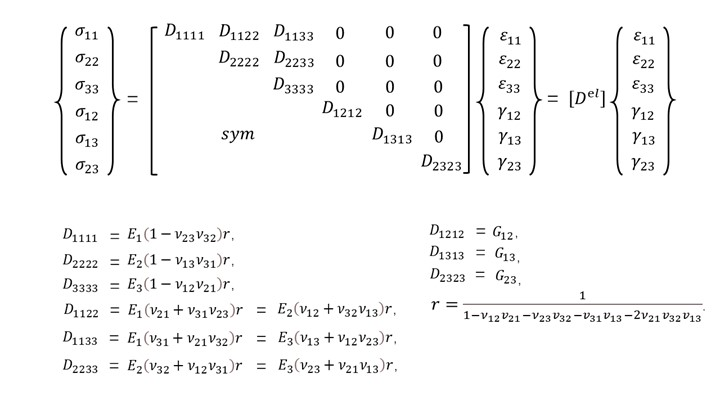Fig. 3. Orthotropic constitutive model of wood

According to the direction definition of the test model in the coordinate axis (Fig. 4a), the model was subjected to vertical monotonic static loading (Chen et al. 2014) in the Z-axis direction and was subjected to horizontal low-cycle reciprocating loading (Fujita et al. 2001; Meng et al. 2018) in the Y-axis and X-axis directions.

Quasi-static test under horizontal low-cycle reciprocating loading: The displacement method was used to control the loading. The first stage was divided into five single reciprocating loads and the amplitude gradually increased. The amplitudes were 0.0125Δ, 0.025Δ, 0.05Δ, 0.075Δ, and 0.1Δ, respectively, where Δ = 50 mm; the second stage started from 0.2Δ, each given amplitude performed three reciprocating loading cycles, and the given amplitude increased by 0.2Δ each time (Cao et al. 2021). The loading protocol is shown in Fig.4b. Setting of the solver, the construction of the connection relationship and the boundary conditions in the simulation are based on the test conditions of the Z axis, Y axis, and X axis.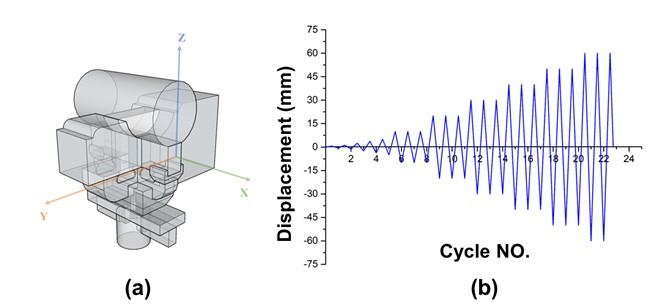Fig. 4. The direction definition of the test model in the coordinate axis and its loading protocol

Grid System

The construction of grid system adopted the second-order grid unit, and the arrangement was hexahedron combined with tetrahedron. The regular parts adopted hexahedron grid, and the complex parts adopted tetrahedron grid. The grid system of Dou-Gong bracket after division and assembly is shown in Fig. 5.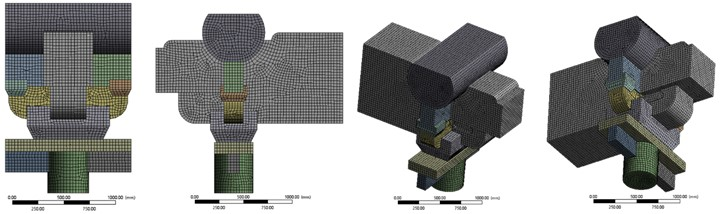Fig. 5. Division of Dou-Gong bracket’s grid system in simulation

RESULTS AND DISCUSSION

The load-displacement curve obtained by the vertical monotonic loading simulation in the Z-axis direction is shown in Fig. 6. The bearing capacity of Dou-Gong bracket model obtained by the simulation does not converge after 337.5 kN. The equivalent stress cloud diagram (Fig. 7A) of the vertical monotonic loading of Dou-Gong bracket in the Z-axis direction is mainly distributed at the mortise and tenon joint of the center and the middle of the component. The maximum stress point is 8.90 MPa, which is located at the dovetail mortise and tenon joint of E-Fang and column cap. The equivalent elastic strain cloud diagram (Fig. 7B) is similar to the distribution of the equivalent stress cloud diagram. The maximum point is 0.014, which is located at the dovetail tenon joint between the left E-Fang and column cap. The strain energy (Fig. 7C) is distributed at the mortise-tenon joint and the column cap, indicating that Dou-Gong bracket effectively transfers the upper energy to the column. The maximum point is 245.3 MJ, which is located at the dovetail mortise-tenon joint between the E-Fang and column cap. The overall deformation (Fig. 7D) gradually decreases from the central truss downwards, with a maximum point of 3.04 mm at the top of the central truss.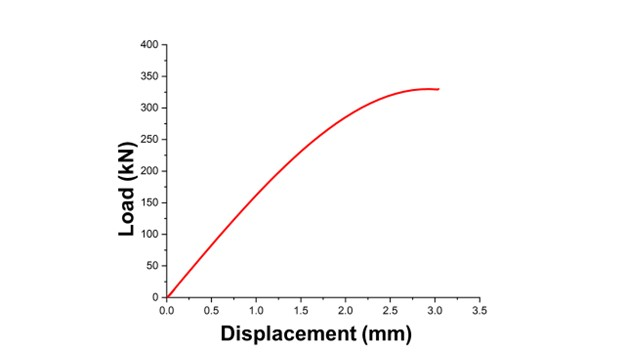Fig. 6. The load-displacement curve in Z-axis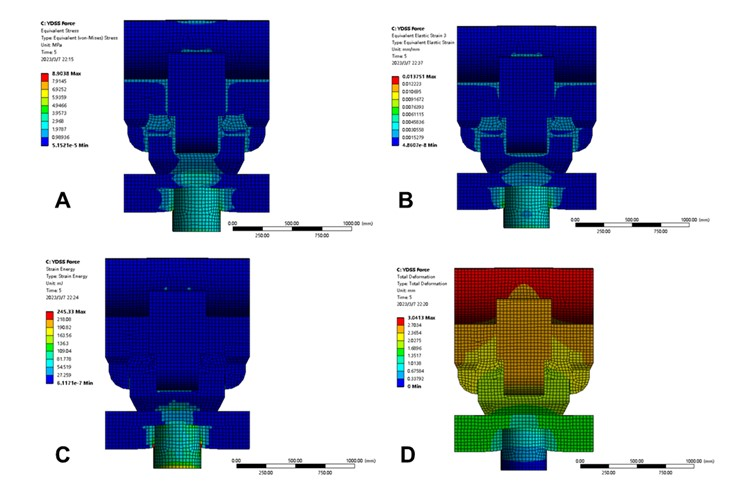Fig. 7. The equivalent stress cloud diagram, equivalent elastic strain cloud diagram, strain energy cloud diagram and overall deformation cloud diagram in Z-axis

The simulation results of the load-displacement hysteresis curve of the quasi-static loading under the horizontal low-cycle reciprocating load in the Y-axis and X-axis directions are shown in Fig. 8. The maximum horizontal thrust of the test model along the Y-axis is 243.6 kN; the maximum horizontal thrust of the test model along the X-axis is 189.4 kN. The hysteresis curves of Dou-Gong bracket on the Y axis and the X axis are both spindle-shaped, reflecting that the plastic deformation ability of the whole component on the Y axis and the X axis is very strong, and it has good seismic performance and energy dissipation capacity. Wood members with a spindle-shaped hysteretic curve are not prone to shear failure when subjected to bending, shearing and compression, for they have good plastic deformation ability because of good ductility and large equivalent viscous damping coefficient.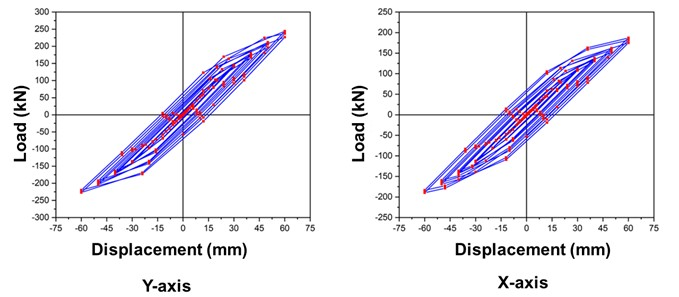Fig. 8. The load-displacement hysteretic curves in Y-axis and X-axis

According to hysteretic curve, the load-displacement skeleton curve can be obtained (Fig. 9), and the stiffness degradation curve of the specimen is obtained by extracting the stiffness of each section of the skeleton curve (Fig. 10).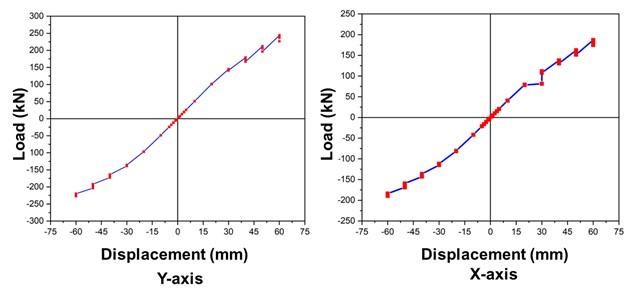Fig. 9. The load-displacement skeleton curves in Y-axis and X-axis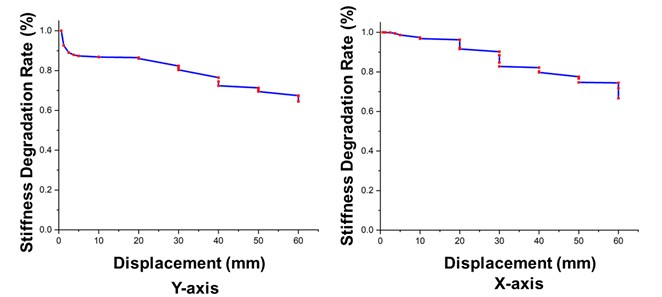Fig. 10. The stiffness degradation curves in Y-axis and X-axis

The equivalent stress cloud diagram (Fig. 11A) of the quasi-static loading of the simulation model under the horizontal low-cycle reciprocating load in the Y-axis direction is mainly distributed at the mortise-tenon joint of the cross between Baotou beam and the central melon arch and the column cap. The maximum stress value is 39.2 MPa, which is located at the mortise-tenon joint of the cross between Baotou beam and the central melon arch. The equivalent elastic strain cloud diagram (Fig. 11B) is similar to the distribution of the equivalent stress cloud diagram, and the maximum value is 0.0488, which is located at the mortise and tenon joint of the cross between Baotou beam and the central melon arch. The strain energy cloud diagram (Fig. 11C) is similar to the stress cloud diagram distribution, indicating that Dou-Gong bracket effectively transfers the upper energy to the column, with a maximum value of 8.818e5 MJ, located on the column cap. The overall deformation cloud diagram (Fig. 11D) is mainly distributed in the mortise and tenon joint and the column cap at the cross of Baotou beam and the central melon arch. The maximum value is 31.9 mm, which is located on the column cap.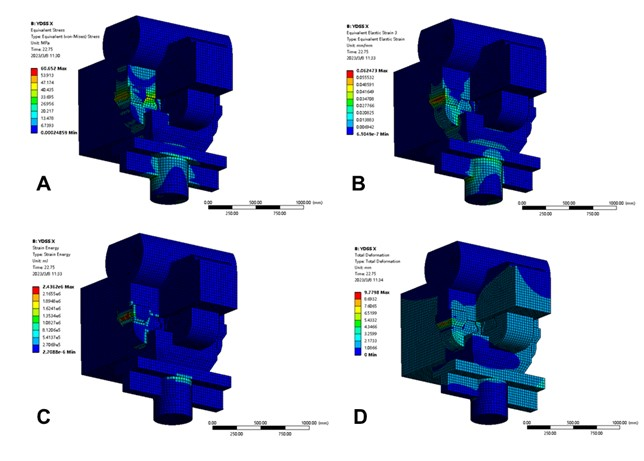Fig. 11. The equivalent stress cloud diagram, equivalent elastic strain cloud diagram, strain energy cloud diagram and overall deformation cloud diagram in Y-axis

The equivalent stress cloud diagram (Fig. 12A) of the quasi-static loading of the simulation model under the horizontal low-cycle reciprocating load in the X-axis direction is mainly distributed at the mortise-tenon joint of the cross between Zhengxin Fang and groove lifter, the central melon arch and the column cap. The maximum stress value is 60.65 MPa, which is located at the mortise-tenon joint at the junction of Zhengxin Fang and the groove lifter. The equivalent elastic strain cloud diagram (Fig. 12B) is similar to the distribution of the stress cloud diagram, with a maximum value of 0.0624, which is located at the mortise and tenon joint at the junction of the upright Zhengxin Fang and groove lifter. The strain energy cloud diagram (Fig. 12C) is similar to the distribution of the stress cloud diagram. The maximum value is 2.436e6 MJ, which is located at the mortise and tenon joint at the junction of Zhengxin Fang and the groove lifter. The overall deformation (Fig. 12D) is widely distributed in the middle of the overall component, with a maximum value of 9.78 mm, which is located at the mortise and tenon joint at the junction of Zhengxin Fang and the groove lifter. The quasi-static loading under the horizontal low-cycle reciprocating load in the X-axis direction may cause the maximum deformation or even damage to the mortise-tenon joint at the junction of Zhengxin Fang and the groove lifter.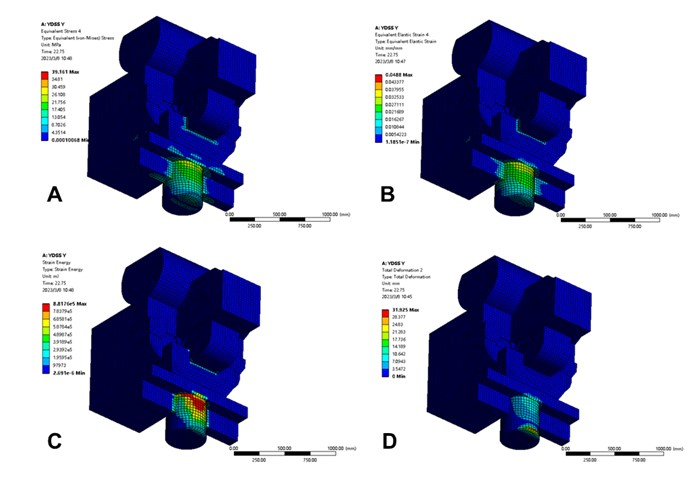Fig. 12. The equivalent stress cloud diagram, equivalent elastic strain cloud diagram, strain energy cloud diagram and overall deformation cloud diagram in X-axis

Mechanical Model of Static Structural Behavior (Z-axis, Y-axis and X-axis)

The mechanical behavior of Qing-style ‘one bucket three liters’ Column-cap Dou-Gong Bracket test model under vertical monotonic static load (Z-axis) conforms to the variable stiffness linear elastic mechanical model (Fig. 13). In the OA stage, the gap between the components of Dou-Gong Bracket is squeezed, and the whole component is subjected to a small amount of load. There is a linear relationship between force and displacement in AB stage. In the BC stage, the structure yielded and the stiffness became degraded. The characteristic points A, B, and C correspond to the squeezing, yield point, and ultimate bearing capacity point of Dou-Gong Bracket, respectively. The calculation of deformation stiffness in OA stage, AB stage, and BC stage is based on Eqs. 1, 2, and 3, respectively.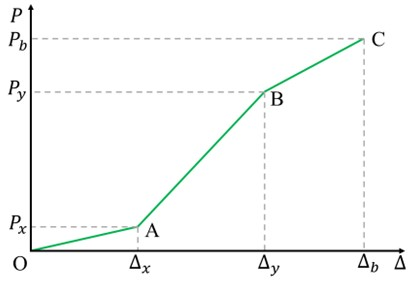Fig. 13. The variable stiffness linear elastic mechanical model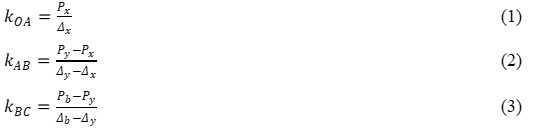According to the simulation results, the deformation stiffness KOA, KAB, and KBC of the three stages of Qing-style ‘one bucket three liters’ Column-cap Dou-Gong Bracket test model under the vertical monotonic static load is 2.61, 7.23, and 3.38 kN/mm, respectively.

According to the hysteresis curve and skeleton curve, the restoring force model (Fig. 14) of the static structural behavior of Qing-style ‘one bucket three liters’ Column-cap Dou-Gong Bracket test model on the Y axis and X axis is established.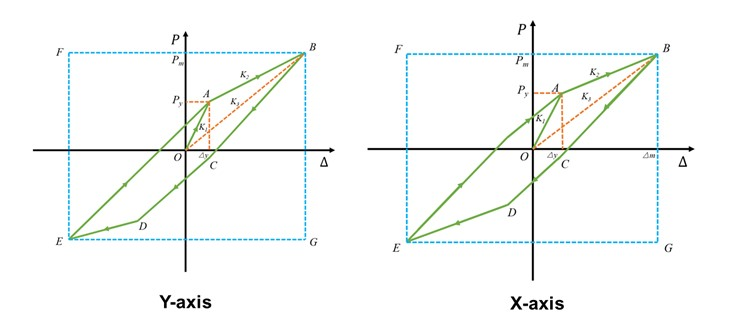Fig. 14. The restoring force model of the static structural behavior in Y-axis and X-axis

On the Y axis, OA is the elastic stage, the displacement of the test model is proportional to the load, and the stiffness KY1 is 10.33 kN/mm; AB is the yield stage, from point A to point B, the stiffness KY2 of the test model in the plastic stage is 2.58 kN/mm; KY3 is the ratio of the maximum bearing capacity to the maximum displacement of the restoring force model, which is called the effective stiffness of Dou-Gong bracket. The effective stiffness KY3 of the test model is 4.13 kN/mm. The static structural behavior of Dou-Gong bracket test model on the X-axis is similar to that of the Y-axis. The stiffness KX1 of OA in the elastic stage, the stiffness KX2 of AB in the yield stage, and the effective stiffness KX3 are 7.32, 2.22, and 3.33 kN mm, respectively.

If the Dou-Gong bracket component is regarded as the shock absorber between the roof and the column of the ancient Chinese timber structure, the nonlinear coefficient NL can be introduced to quantitatively evaluate the damping effect. NL is equal to the ratio of the envelope area of the restoring force model to the rectangular area SBGEF in Fig. 14. NL can characterize the energy dissipation capacity of Dou-Gong bracket component. The nonlinear coefficient NL of Qing-style ‘one bucket three liters’ Column-cap Dou-Gong Bracket test model in the Y-axis direction is 0.187, and the nonlinear coefficient NL in the X-axis direction is 0.207.

The ratio of ultimate displacement and yield displacement of Dou-Gong bracket is the ductility of the component, and the greater ductility reflects that the component has better deformation ability. The ductility of Qing-style ‘one bucket three liters’ Column-cap Dou-Gong Bracket test model in the Y-axis direction is 5.23, and the ductility in the X-axis direction is 4.57 according to the simulation results.

The larger the equivalent viscous damping coefficient (he) is, the stronger the energy dissipation capacity of Dou-Gong bracket component is. The equivalent viscous damping coefficient is calculated according to Fig. 15 and Eq. 4. The equivalent viscous damping coefficient of Qing-style ‘one bucket three liters’ Column-cap Dou-Gong Bracket test model in the Y-axis direction is 0.104, and the equivalent viscous damping coefficient in the X-axis direction is 0.121.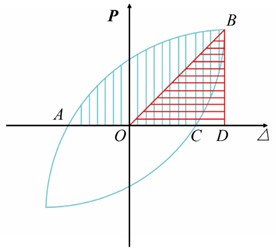Fig. 15. Calculation of equivalent viscous damping coefficient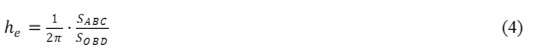From the three directions of the X, Y, and Z axes, the static structural behavior and stress deformation characteristics of Qing-style ‘one bucket three liters’ Column-cap Dou-Gong Bracket test model are evaluated as follows: The structural behavior under vertical monotonic static loading in the Z-axis direction conforms to the variable stiffness linear elastic mechanics model, and its variation characteristics are divided into three stages. In the first stage, the gaps and nodes between the components are compacted. In the second stage, the structure is in the linear stage, and the load and displacement change linearly. In the third stage, the structure yields until it is destroyed. The structural behavior under horizontal low-cycle reciprocating load in the Y and Z axis conforms to the multi-linear restoring force model, and its variation characteristics are divided into three stages: elasticity, yield and failure. During the loading process, the stiffness of Dou-Gong bracket gradually decreases, and the hysteresis loop near the origin of the coordinate pinches up, showing slip characteristics. After the horizontal load increases to a certain extent, the component undergoes severe plastic deformation and the displacement increases sharply. The key mechanical indexes obtained from the three aspects of strength, deformation and energy to evaluate the static performance of Qing-style ‘one bucket three liters’ Column-cap Dou-Gong Bracket test model is shown in Table 2.

Table 2. Characteristic Values of the Six Specimen Configurations